Sunday 25th July 2021# Classroom Key Stage 2 / KS2 - Maths Activities & Resources

## Welcome to the Maths section of the Key Stage 2 classroom. Within KS2 Maths you will find online @school assessment tests that will help children to enhance their learning and revise the subject of Maths at Key Stage 2 level.

•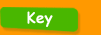•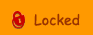••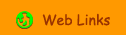•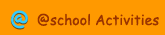•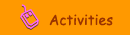•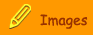###Subtraction

Adding and Subtracting - Year 3 - On line activity about completing various adding and subtracting sums.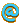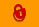Maths Generator - Create online sums either online or as a worksheet including addition, subtraction, multiplication and division.Reverse Addition - Interactive activity to use the relationship between addition and subtractionSubtract 11 - Interactive activity to subtract 11Subtract 9 - Interactive activity to subtract 9Subtract a 2-Digit Number - Interactive activity to learn how to use partitioning to subtract 2-digit numbers.Subtraction  Year 3 - Twenty-two interactive or printable subtrction activitiesSubtraction  Year 4 - Eight ineteractive or printable activities for subtractionSubtraction - Year 5 - Ten interactive or printable activities for subtractionSubtraction  Year 6 - Four interactive or printable activities for subtractionSubtraction Sums - Hard - 1 - Interactive activity about hard subtraction sums.Subtraction up to 20 - Interactive activity using number grid up to 20Subtraction up to 40 - Interactive activity using number grid up to 40Subtraction Using Negative Numbers - Interactive activity using number gridSum Sense - Subtraction - Interactive activity about subtractionWritten sums - Year 4 - Interactive activity about written sums.